Visualizing Support Vector Machine (SVM)

Support Vector Machine is a Supervised machine learning Algorithm used for performing classification as well as regression related tasks. It is a popular choice among the machine learning experts due to its high accuracy and optimization and today we will understand the reason behind it.

Note that the whole blog would be focused only on the geometric intuition of SVM in order to provide a better understanding to the readers.

## Expected Questions to be answered in this blog:

1. What are Support Vectors?

2. How Support Vector Classifier works?

3. What makes it different from Logistic and Linear Regression models?

4. How does a Support Vector Machine works?

# Support Vectors

Consider the image below,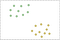fig 3.1 Datapoints belonging to two different class

There is a group of points belonging to 2 different classes. Our aim is to find a hyperplane( line in 2D ) that divides these two classes with maximum distance possible between the line and data points.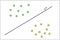fig 3.2

We get this figure, Assume that the points above the line are positive and those below the line are negative.

Now we draw a dotted line w’ parallel to w intersecting our nearest occurring positive data-points. Similarly, we draw another dotted line w’’ parallel to w intersecting our nearest occurring negative data-points.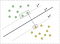fig 3.3 Datapoints enclosed within boxes are called Support vectors

The distance between w’ and w’’ is known as the Marginal distance and our main goal is to maximize this distance as much as possible

## So now coming to the Support Vectors, The data-points intersected by w’ and w’’ are called Support Vectors. In fig 3.3, the data-points enclosed within the box are the Support Vectors.

Support Vectors are very important as they influence the orientation of the hyperplane. They are also used to calculate the Marginal distance.

# Hard Margin Classifier

In order to understand the Support Vector Classifier, we must first understand what is a hard margin classifier and what is its drawback.

Here, You can notice that the points which lie above w’ are positive and those lie below w’’ are negative. This type of classification does not allow points to lie within the margin. So they are called Hard Margin Classier.

This condition gets worse when we have an outlier in the dataset. Consider the image below,

Here, there is a data point below our decision boundary w which is labeled as positive. Now if we go by the rules of a Hard Margin classifier. We will draw a separating line like this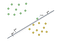fig 3.6

Because of this, our Decision boundary (w) is greatly impacted. This condition is called Over-fitting. To understand even better Imagine in our test dataset we have 5 negatively labeled points near the outlier. Now all these 5 points will be miss-classified by our classifier as positive because of that one outlier the whole line(w) is shifted.

# Support Vector Classifier

This Classifier amends the drawbacks of the Hard Margin classifier by allowing some miss-classification without hugely affecting the line W.

Consider the above figure (fig 3.6) again, In this scenario, the Support vector classifier would allow the outlier point and starts calculating the marginal distance from the next nearest negative data-point. Thereby, doing better classification at validation.

Now, You might be thinking what if there are multiple miss-classified points in the dataset

Here the classifier itself undergoes through a process of cross-validation in other words trying each combination and finding the optimal soft-margin resulting in better classification.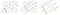Finding the best marginal distance by cross-validation

How SVM classification is different from Logistic Regression?

Best I can explain this by saying that Logistic Regression finds the best-fit line/ Hyperplane with the help of all the data points whereas SVM finds the Hyper-plane with the help of only the Support vectors. So with logistic regression, you do not get a strict hyperplane separating linearly-separable points with maximum distance possible.

# Support Vector Machines

It is a little amendment to the Soft-margin classifier/Support Vector Classifier. But trust me the result is overwhelming. Before going into the depth let us see some problems SVMs can solve.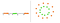Left: 1-Dimension Figure Right: 2-Dimension Figure

If you try to classify these data-points with Logistic Regression or Soft-margin classifier. You will likely achieve around 50% accuracy because this type of data is not linearly separable.

The way SVM handles this is by converting the lower dimension dataset into a higher dimension.

Consider the example above in 1-D(left), Here the points are only classified as orange if they belong between the range of 0 - 10 and above 30 else they are classified as green. Now what SVM does is it applies a function to each of the data-point.

Let the function be

If we apply this function to each data-point. The figure will look like this

Now we can easily divide these points by a Line W.

Similarly consider the example in 2-D. Here, SVM solves this by computing it into 3-D, such that the points look like this,

Now we can easily classify these points by drawing a plane.

All of these transformations take place by a special unit in SVM known as Kernels. Kernal contains the set of mathematical functions that are required to transform non-linear problems into higher dimensions. Kernal functions do not transform the data points into higher dimensions instead they calculate the relationship as if they were in higher dimensions.

Keep Learning! :)

A Machine Learning Junkie

## More from Aditya Tiwari

A Machine Learning Junkie